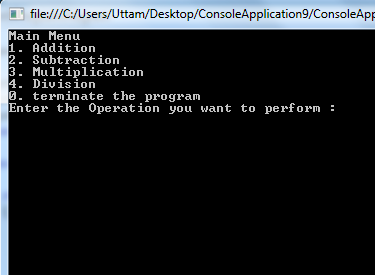C# is a simple, modern, general-purpose, object-oriented programming language. Making Calculator is one of the basic program by which we can learn and revise many concept. An arithmetic operator is a symbol that tells the compiler to perform specific mathematical operation.

So, I have decided to make a calculator by using switch and do while case. Below are some syntaxes which I have used in the program:

Console & Convert: They are the pre defined classes into the system.

Convert.ToInt32: It is used to convert the string into integer.

Console.WriteLine(): This syntax is used for output of the program or any useful relevant information.

Console.ReadLine(): This syntax is used to take the input from the user side.

##### Here is the complete code:
`using System;using System.Collections.Generic;using System.Text;namespace Program{    class Program    {        static void Main(string[] args)        {            int Num1 = 0, Num2 = 0, result, opt=0;            do            {                Console.WriteLine("Main Menu");                Console.WriteLine("1. Addition");                Console.WriteLine("2. Subtraction");                Console.WriteLine("3. Multiplication");                Console.WriteLine("4. Division");                Console.WriteLine("0. terminate the program");                 Console.Write("Enter the Operation you want to perform : ");                opt = Convert.ToInt32(Console.ReadLine());                                if (opt == 4)                    Console.WriteLine("Please don't enter 2nd number as zero");                 if (opt >= 1 && opt <= 4)                {                    Console.Write("Enter the First Number : ");                    Num1 = Convert.ToInt32(Console.ReadLine());                     Console.Write("Enter the Second Number : ");                    Num2 = Convert.ToInt32(Console.ReadLine());                    if (opt == 4 && Num2 == 0)                        Console.WriteLine("Not possible");                }                 switch (opt)                {                    case 1:                        result = Num1 + Num2;                        Console.WriteLine("Addtion of {0} & {1} is equal to  {2}\n",                                                    Num1, Num2, result);                        break;                    case 2:                        result = Num1 - Num2;                        Console.WriteLine("Subtraction of {0} & {1} is equal to  {2}\n",                                                     Num1, Num2, result);                        break;                    case 3:                        result = Num1 * Num2;                        Console.WriteLine("Multiplication of {0} & {1} is equal to  {2}\n",                                                     Num1, Num2, result);                        break;                    case 4:                        if (Num2 == 0)                            break;                        result = Num1 / Num2;                        Console.WriteLine("Division of {0} & {1} is equal to  {2}\n",                                                     Num1, Num2, result);                        break;                    default:                        if (opt != 0)                            Console.WriteLine("Invalid option \n");                        else                            Console.WriteLine("End of the program \n ");                         break;                }            } while (opt != 0);            Console.ReadLine();        }    }}`

##### Output:Modified On Mar-16-2018 05:49:40 AM
1.Thanks Abhishek it is very informative post about buliding a calculator